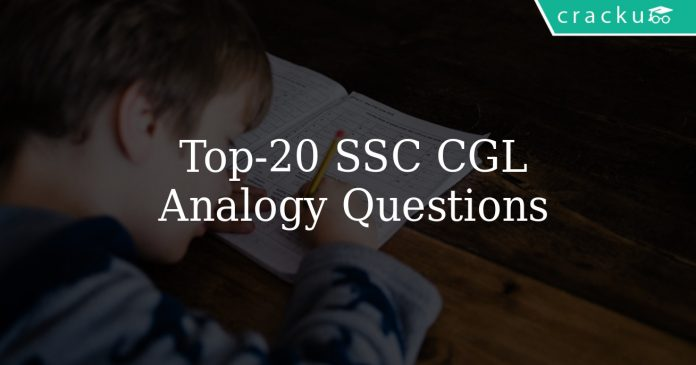# Top-20 SSC CGL Analogy Questions

0
260Top-20 SSC CGL Analogy Questions

# SSC CGL Analogy Questions:

Download Top-20 Analogy questions for SSC CGL exam. Most important Analogy  questions based on asked questions in previous exam papers for SSC CGL.

Instructions

In each of the following questions, select the related word/letters/number from the given alternatives.

Question 1: Hot : Cold : : ?

a) Summer : Sun

b) Germs : Disease

c) Light : Dark

d) Thin : Big

Instructions

In the following six questions, select the related word/letters/number from the given alternatives.

Question 2: AEFJ : KOPT : ? : QUVZ

a) HKQL

b) GLKP

c) GKLP

d) HLKP

Question 3: 2 : 32 :: 3 : ?

a) 143

b) 243

c) 293

d) 183

Question 4: D × H : 4 × 8 , as M × Q : ?

a) 14 × 18

b) 12 × 17

c) 12 × 16

d) 13 × 17

Question 5: Haematology : Blood : Phycology : ?

a) Diseases

b) Fungi

c) Fishes

d) Algae

Question 6: Pride of Lions : : _______ of Cats

a) Bunch

b) Herd

c) School

d) Clowder

Question 7: MAN : PDQ : : WAN : ?

a) YDQ

b) ZDQ

c) NAW

d) YQD

Instructions

In the following six questions, find the odd word/number/letters/number pair from the given alternatives.

Question 8:

a) GFHJ

b) CBEF

c) EDGH

d) IHKL

Question 9:

a) 5061

b) 4025

c) 7202

d) 6023

Question 10:

a) 104 : 78

b) 96 : 80

c) 64 : 48

d) 80 : 60

Question 11:

a) Night

b) Morning

c) Noon

d) Evening

Question 12:

a) Fraternity

b) Liberty

c) Society

d) Equality

Question 13:

a) KQNN

b) DWFU

c) EVHS

d) HSKP

Question 14: In a certain code “MOUSE” is written as “PRUQC”. How is “SHIFT” written in that code?

a) VKIDR

b) VJIDR

c) VIKRD

d) RK.IVD

Question 15: Name a single letter, which can be prefixed to the following words in order to obtain entirely new words ?
TILL TABLE PILE TAB PRING

a) C

b) S

c) B

d) H

Instructions

In the following questions, select the related word/letters/number from the given alternatives.

Question 16: $\sqrt{AFI}=M:\sqrt{ADD}=L::\sqrt{ABA}$ ?

a) I

b) N

c) O

d) K

Question 17: 16 : 22 : : 36 : ?

a) 44

b) 24

c) 26

d) 46

Question 18: PALAEONTOLOGY : FOSSIL : : PHRENOLOGY : ?

a) SKULL

b) THYROID

c) PANCREAS

d) LUNGS

Question 19: 9 : 50 : : ?

a) 22 : 110

b) 18 : 190

c) 15 : 225

d) 20 : 105

Question 20: MIKE : OGMC : CIAD : ?

a) EGCB

b) EICB

c) AJCF

d) ENCF

Answers & Solutions:

1) Answer (C)

Hot and cold are antonyms, among the options, words which are opposite of each other are

= Light : Dark

=> Ans – (C)

2) Answer (C)

Expression = AEFJ : KOPT

The pattern followed is :

Similarly, for QUVZ :

=> Ans – (C)

3) Answer (B)

Expression = 2 : 32 :: 3 : ?

The pattern followed is = $n:n^5$

Eg = $2:2^5=2:32$

Similarly, $3^5=243$

=> Ans – (B)

4) Answer (D)

Given = D × H : 4 × 8

Alphabets in English alphabetical series are given numbers starting from A = 1 , B = 2 , C = 3 and so on.

Similarly, M = 13 and Q = 17

Thus, M × Q : 13 × 17

=> Ans – (D)

5) Answer (D)

Haematology is the branch of medicine involving study and treatment of the blood. Similarly, Phycology is the branch of botany concerned with seaweeds and other algae.

=> Ans – (D)

6) Answer (D)

A group of lions is called Pride of Lions, similarly Clowder of cats is a group of cats.

=> Ans – (D)

7) Answer (B)

Expression = MAN : PDQ

The pattern followed is =

Similarly, for WAN :

=> Ans – (B)

8) Answer (A)

(A) : G (-1 letter) = F (+2 letters) = H (+2 letters) = J

(B) : C (-1 letter) = B (+3 letters) = E (+1 letters) = F

(C) : E (-1 letter) = D (+3 letters) = G (+1 letters) = H

(D) : I (-1 letter) = H (+3 letters) = K (+1 letters) = L

=> Ans – (A)

9) Answer (A)

The sum of digits of each number :

(A) : 5061 = 5 + 0 + 6 + 1 = 12

(B) : 4025 = 4 + 0 + 2 + 5 = 11

(C) : 7202 = 7 + 2 + 0 + 2 = 11

(D) : 6023 = 6 + 0 + 2 + 3 = 11

=> Ans – (A)

10) Answer (B)

The ratio of each number is :

(A) = 104 : 78 = $\frac{4}{3}$

(B) = 96 : 80 = $\frac{6}{5}$

(C) = 64 : 48 = $\frac{4}{3}$

(D) = 80 : 60 = $\frac{4}{3}$

=> Ans – (B)

11) Answer (C)

Night, morning and evening are periods of a day, while noon is a time (12 pm).

=> Ans – (C)

12) Answer (C)

Liberty, Equality and Fraternity are all ideals, hence society is the odd one out.

=> Ans – (C)

13) Answer (B)

If we combine the first and third and fourth and second letters in each word in that order, we find that there is a gap of 3 letters in between.

(A) : K (+3 letters) = N , N (+3 letters) = Q

(B) : D (+2 letters) = F , U (+2 letters) = W

(C) : E (+3 letters) = H , S (+3 letters) = V

(D) : H (+3 letters) = K , P (+3 letters) = S

=> Ans – (B)

14) Answer (A)

“MOUSE” is written as “PRUQC”

The pattern followed is :

Similarly, for SHIFT :

=> Ans – (A)

15) Answer (B)

Words : TILL TABLE PILE TAB PRING

If ‘S’ is prefixed to each of the word above, new words will be :

STILL , STABLE , SPILE , STAB , SPRING

=> Ans – (B)

16) Answer (D)

If we assign numbers to the alphabets such as A = 1 , B = 2 , C = 3 and so on, we get :

$\sqrt{AFI}=\sqrt{1+6+9}=\sqrt{16}=4=1+3\equiv13=M$

and $\sqrt{ADD}=\sqrt{1+4+4}=\sqrt{9}=3=1+2\equiv12=L$

Similarly, $\sqrt{ABA}=\sqrt{1+2+1}=\sqrt{4}=2=1+1\equiv11=K$

=> Ans – (D)

17) Answer (A)

Expression = 16 : 22 : : 36 : ?

The pattern followed is = $n^2:n^2+n+2$

Eg = $4^2:4^2+4+2=16:22$

Similarly, $36=6^2$

Thus, $6^2+6+2=36+8=44$

=> Ans – (A)

18) Answer (A)

Palaeontology is the branch of science concerned with fossil, similarly Phrenology is the study of skull.

=> Ans – (A)

19) Answer (D)

Expression = 9 : 50 : : ?

The pattern followed is = $n:5n+5$

Eg = $9:5(9)+5=9:50$

Similarly, $20(5)+5=100+5=105$

=> Ans – (D)

20) Answer (A)

Expression = MIKE : OGMC

The pattern followed is =

Similarly, for CIAD :

=> Ans – (A)